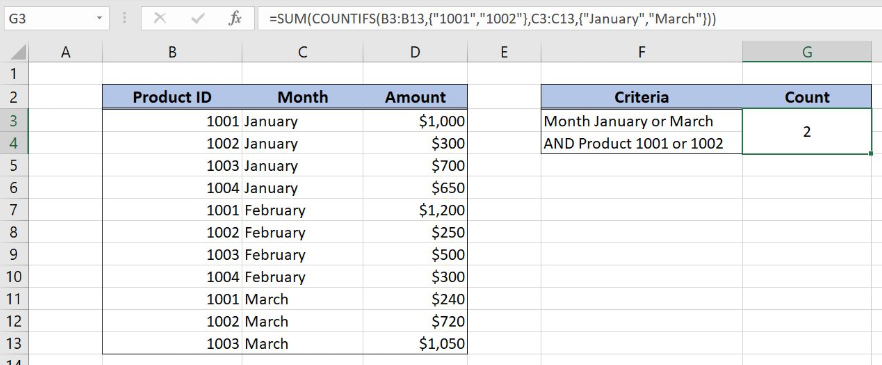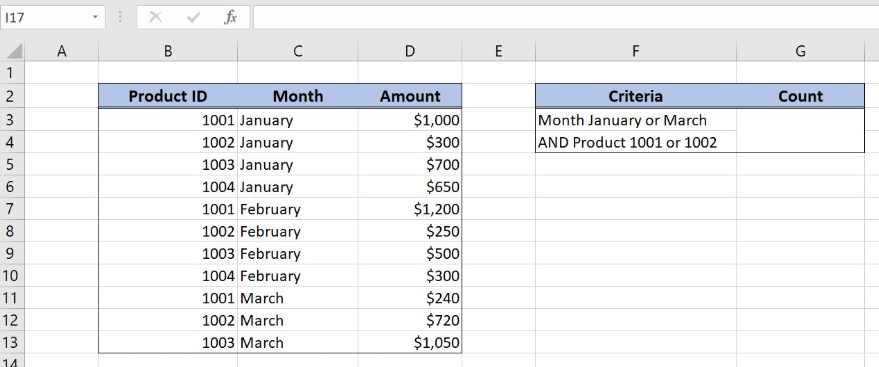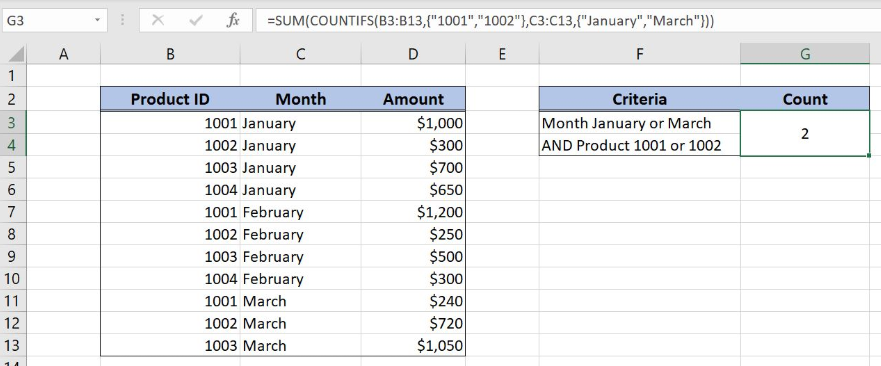Get instant live expert help with Excel or Google Sheets“My Excelchat expert helped me in less than 20 minutes, saving me what would have been 5 hours of work!”

#### Post your problem and you’ll get Expert help in seconds.

Your message must be at least 40 characters
Our professional Expert are available now. Your privacy is guaranteed.

# How to Use COUNTIFS with Multiple Criteria and OR Logic

Excel allows a user to count values with multiple criteria and or logic using the COUNTIFS and SUM functions. This step by step tutorial will assist all levels of Excel users in creating a COUNTIFS with multiple criteria and or logic.Figure 1. The result of the formula

## Syntax of the COUNTIFS Formula

The generic formula for the COUNTIFS function is:

`=COUNTIFS(criteria_range1, criteria1, criteria_range2, criteria2, ... )`

The parameters of the COUNTIFS function are:

• criteria_range1, criteria_range2 – ranges where we want to apply our criteria
• criteria1, criteria2 – a criteria in criteria ranges which we want to count.

## Syntax of the SUM Formula

The generic formula for the SUM function is:

`=SUM(number1, number2, …, numberN)`

The parameters of the SUM function are:

• number1, number2, …, numberN – numbers which we want to sum.

## Setting up Our Data for the Formula

In the first data table, we have 3 columns: “Product ID” (column B), “Month” (column C) and “Amount” (column D). In the cells F3 and F4 we have criteria for counting. We want to count items which have the month January or March and Product ID 1001 or 1002. The results will be in the cell G3.Figure 2. Data that we will use in the example

## Count Items with Multiple Criteria and Or Logic

In our example, we want to count items which have the month January or March and Product ID 1001 or 1002.

The formula looks like:

`=SUM(COUNTIFS(B3:B13, {"1001", "1002"}, C3:C13, {"January", "March"}))`

The parameter criteria_range1 is B3:B13 and the criteria1 is the array  {“1001”, “1002”}. The parameter criteria_range2 is C3:C13 and the criteria2 is {“January”, “March”}. The result of this COUNTIFS function is 2 numbers, which are number parameters of the SUM function.

To apply the formula, we need to follow these steps:

• Select cell G3 and click on it
• Insert the formula: `=SUM(COUNTIFS(B3:B13,{"1001","1002"},C3:C13,{"January","March"}))`
• Press enter.Figure 3. Counting items with multiple criteria and or logic

As you can see, we have two items meeting both criteria. In row 3, the Product ID is 1001 and the month is January and in row 12 we have the Product ID 1002 and the month March. Therefore, the SUM function sums these two items and the result in G3 is 2.

Most of the time, the problem you will need to solve will be more complex than a simple application of a formula or function. If you want to save hours of research and frustration, try our live Excelchat service! Our Excel Experts are available 24/7 to answer any Excel question you may have. We guarantee a connection within 30 seconds and a customized solution within 20 minutes.

### Did this post not answer your question? Get a solution from connecting with the expert.Another blog reader asked this question today on Excelchat:
Solution examplesI need a formula to pop at the upper left corner of a spreadsheet. If I enter the month "January," I want the column number sum of January =SUM(AB11:AB75) from another section on the same excel page to pop right below the "January" cell, and not display the formula expression, but see the \$100.
Solved by T. Q. in 40 minsCan't add (SUM) in imported numbers from bank account
Solved by F. C. in 40 minsI need a formula to combine D2 to D100 to add together a column of numbers, then take away the same amount on the same row when column E is filled. i.e. column D is a price of an item, so the formula must calculate the total, then when the item is sold an 'a' is marked next to the item in column E, the formula then must deduct this amount from the total
Solved by X. W. in 20 minsI would like to have a diagram in a new sheet, where the horizontal axis is the days, as they are in column DX. Each day shall show the sum of all unique leads of that day, and I would like to be able to check via a box of checkboxes, which facilities are shown, the facilities are in column BC.
Solved by I. A. in 45 minsI am working on a cash flow projection. Part of the projection includes sales commissions. Our sales guys earn a monthly draw and then commission on sales after a certain amount. For example they may earn a monthly salary of 12,500 and earn additional commission after their commission equals \$150,000. What I need excel to do is sum a column if the values in the preceeding columns are greater than \$150,000. I've tried using the sumif and the if function in excel and it's not working correctly either way. Any suggestions?
Solved by G. W. in 19 mins## Subscribe to Excelchat.coAnother blog reader asked this question today on Excelchat: